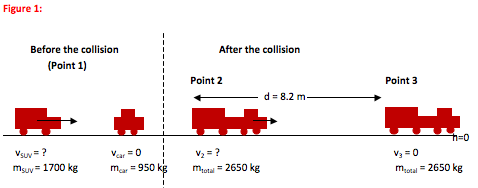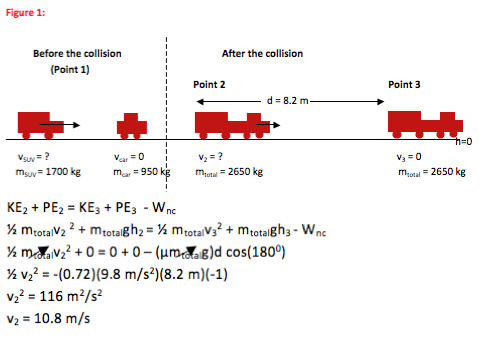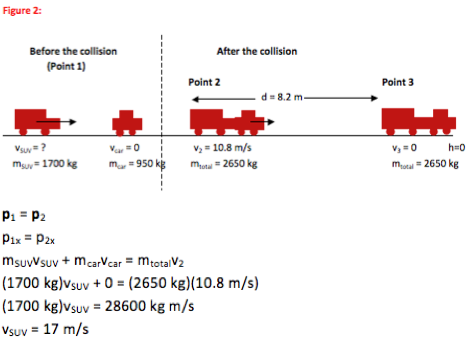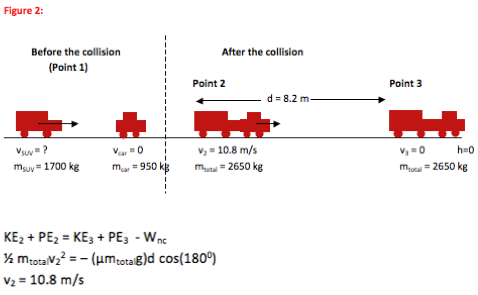# University of Wisconsin Green Bay

The driver of an SUV (m = 1700 kg) isn’t paying attention and rear ends a car (m = 950 kg) on level ground at a red light. On impact, both drivers lock their brakes. The SUV and car stick together and travel a distance of 8.2 m before they come to a stop. How fast was the SUV traveling just before the collision? The coefficient of friction between the tires and the road is 0.72.

• In this problem, you are asked to find the speed of an SUV just before a collision. Any time you are asked to find speed or velocity of an object just before or just after a collision or separation, you should check to see if you can use Conservation of Momentum to solve the problem. Here, you are not given speed information just after the collision. Instead, you are given information about how far the car and SUV travel. So this is not a one-step problem.

If non-conservative forces are either known (as is the case with friction in this problem) or small and if energy is converted from one form to another between the locations, then any time you relate speed and position of an object at two different points conservation of energy is the most direct way to understand the problem.

In this case, you are given stopping force and stopping distance. In other words, you know how much work is done by the non-conservative forces, so you can relate that work to the kinetic energy (and therefore velocity) of the car and SUV just after the collision. In other words, energy is, indeed, the most direct way to learn the velocity of the car and SUV just after the collision.

This, then, is a two part problem. Energy can be tracked from just after the collision until the car and SUV come to a stop. Momentum is conserved during the time of the collision. If you do not recognize both parts of the problem before you begin, that is fine. You can start the problem with either momentum or energy and will quickly find that you need to do an additional problem to find that intermediate velocity.

•For Conservation of Momentum problems, you always draw a picture of the system immediately before the collision or separation and another picture immediately after (Points 1 and 2.) Because momentum depends on mass and velocity, label all mass and velocity information on the pictures. This helps to avoid mistakes as you fill into the equation later.

For Conservation of Energy problems, you want to show the velocity and position information at all points over which you track energy (Points 2 and 3.)

•Pb = Pa

Any time you understand the motion of a system for which Fextermal Δt≈0, you begin with the Conservation of Momentum equation.

KE2 + PE2 = KE3 + PE3 – Wnc

Any time you understand the motion of an object by looking at its energy, you begin with the Conservation of Energy equation. This form of the equation works whenever you can track Wnc.

In this problem, we know information about Point 3 and want to learn about Point 1. Therefore, we will begin with the Conservation of Energy equation—we can use information about Point 3 to learn about Point 2. Information about Point 2 is needed to learn about Point 1.

• Step OneAt Point 2, the car/SUV system has enough kinetic energy to travel 8.2 m with the brakes locked. This translates to a speed of 10.8 m/s at Point 2. Continue on to use this information to find the speed of the SUV before the collision.

Step TwoThe SUV was traveling at a speed of 17 m/s before the collision. No further mathematical solution is needed for this problem.

•Although the problem asks you to find the speed of the SUV before the collision, you do not have enough information about the speed of the car/SUV system after the collision to begin with Conservation of Momentum. You first need to use distance and speed information to track the energy after the collision to find this velocity.

The energy chain for this motion is kinetic energy→ heat and sound

or

½ mtotalv22 = – Ffrictiond cos(1800)
as seen in the equation above. The speed of 10.8 m/s (25 mph) is reasonable.

Once we know the speed of the car and SUV immediately after the collision (Point 2), we can use that information in the Conservation of Momentum equation to find the speed of the SUV immediately before the collision.

p1 = p2
mSUVvSUV + mcarvcar = mtotalv2
vSUV = 17 m/s

Again, the value of 17 m/s (39 mph) is physically reasonable.